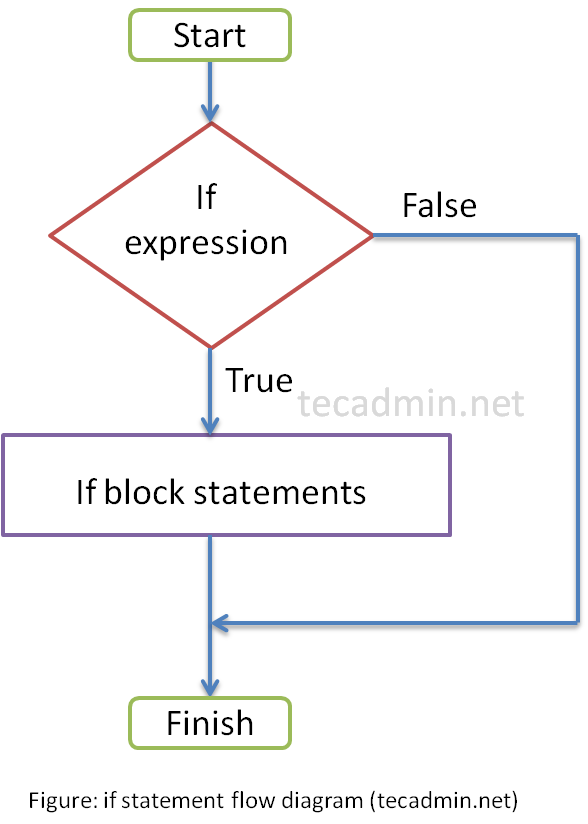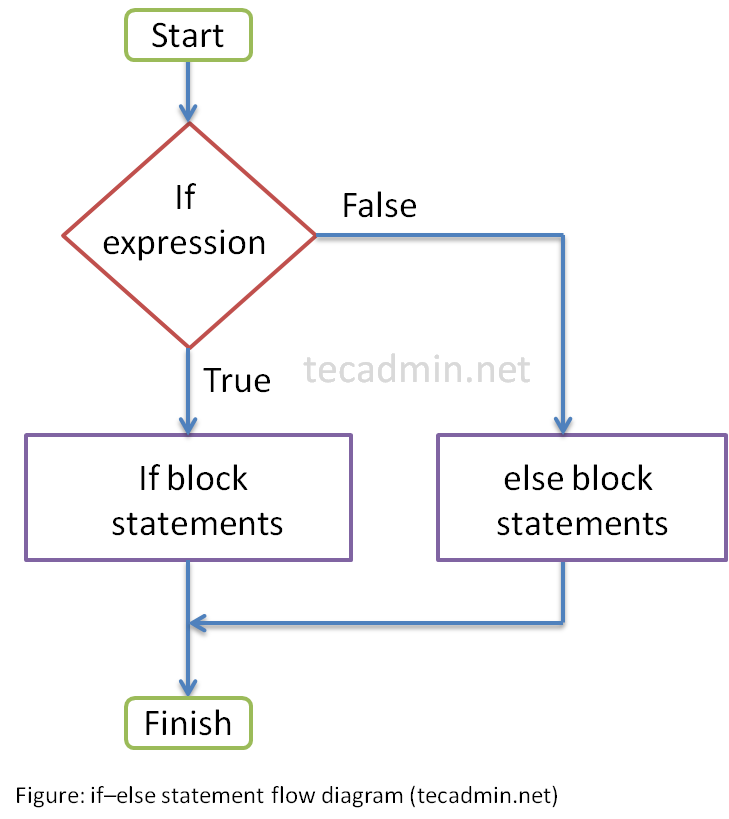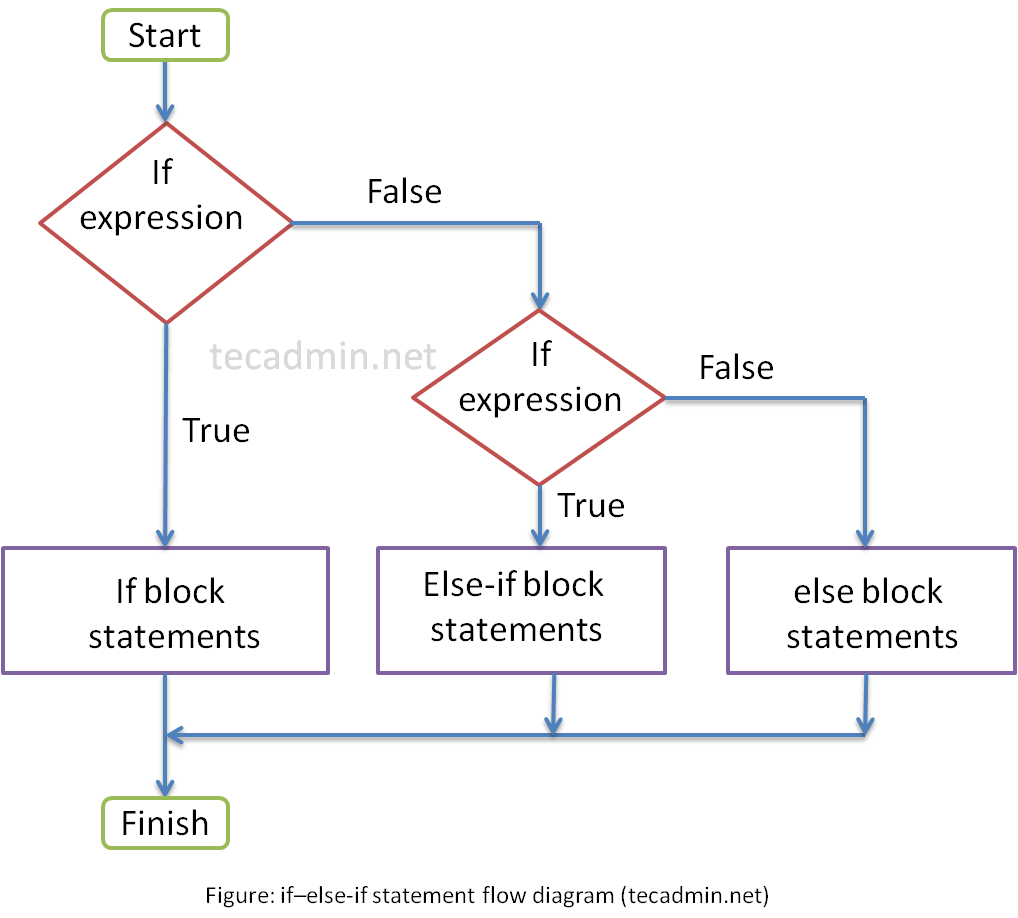1. Home
2. C Programming
3. Control Statements
4. C – if-else

# C – If-Else Statement

The if-else statements are used to run specific code based on condition. The if statement executes a statement if a specified condition is true. If the condition is false, another statement can be executed. Basically, there are 4 types of if statements.

• if statement
• if-else statement
• nested if statement

## 1. if Statement

An if statement evaluates the expression written inside the parenthesis.

```if (Expression)
{
// statement block
}
```

If the expression returns true, The if statement block will be executed. If the expression return false (zero), the statement block will be skipped.Example: if Statement

The following program with input a number from the user and check if the number is less than 10.

## 2. if-else Statement

An if statement evaluates the expression and execute the statements based on results.

```if (Expression)
{
// if statement block
}
else
{
// else statement block
}
```

If the expression returns true, The if statement block will be executed. If the expression return false (zero), the else statement block will be executed.Example: if-else Statement

The following program with input a number from the user and check if the number is less than 10.

## 3. if-else-if Statement

An if statement evaluates the expression and executes the statements based on results.

```if (Expression)
{
// statement 1
}
else if (Expression)
{
// statement 2
}
```

If the expression returns true, The statement 1 block will be executed. If the expression return false (zero), the control go to else and again evaluates a expression.Example: if-else-if Statement

The following program with input a number from the user and check if the number is less than 10.

Tags ,